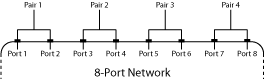# s2sdd

Convert 4-port, single-ended S-parameters to 2-port, differential-mode S-parameters (Sdd)

## Syntax

``sdd_params = s2sdd(s_params)``
``sdd_params = s2sdd(s_params,option)``

## Description

example

````sdd_params = s2sdd(s_params)`converts the 2N-port, single-ended S-parameters to N-port, differential-mode S-parameters. ```
````sdd_params = s2sdd(s_params,option)` converts S-parameters based on the optional `option` argument, which indicates the port-ordering convention of the S-parameters.```

## Examples

collapse all

Create a `sparameters` object from a data file.

`sparams = sparameters('default.s4p');`

Create a data object to store the differential S-parameters.

```diffSparams = rfdata.network; diffSparams.Freq = sparams.Frequencies; diffSparams.Data = s2sdd(sparams.Parameters); diffSparams.Z0 = 2*sparams.Impedance;```

Create a new circuit object with the data from the data object.

```diffCkt = rfckt.passive; diffCkt.NetworkData = diffSparams;```

Analyze the new circuit object.

```frequencyRange = diffCkt.NetworkData.Freq; ZL = 50; ZS = 50; Z0 = diffSparams.Z0; analyze(diffCkt,frequencyRange,ZL,ZS,Z0); diffData = diffCkt.AnalyzedResult;```

Write the differential S-parameters into a Touchstone data file.

`write(diffCkt,'diffsparams.s2p')`
```ans = logical 1 ```

Convert network data to differential-mode S-Parameters using the default port ordering.

```S = sparameters('default.s4p'); s4p = S.Parameters; s_dd = s2sdd(s4p);```

To display differential-mode S-Parameters at the first frequency, type the following command:

`s_dd2 = s_dd(:,:,1)`
```s_dd2 = 2×2 complex -0.0124 - 0.0433i -0.5434 - 0.6872i -0.5428 - 0.6900i -0.0192 - 0.0504i ```

## Input Arguments

collapse all

2N-port single-ended S-Parameters, specified as a complex 2N-by-2N-by-M array, where M represents the number of frequency points of 2N-port single-ended S-Parameters.

Port order, a scalar, specified as `1`, `2`, or `3`. Port order determines how the function orders the ports:

• `1``s2sdd` pairs the odd-numbered ports together first, followed by the even-numbered ports. For example, in a single-ended, 8-port network:

• Ports 1 and 3 become differential-mode pair 1.

• Ports 5 and 7 become differential-mode pair 2.

• Ports 2 and 4 become differential-mode pair 3.

• Ports 6 and 8 become differential-mode pair 4.

The following figure illustrates this convention for an 8-port device.• `2``s2sdd` pairs the input and output ports in ascending order. For example, in a single-ended, 8-port network:

• Ports 1 and 2 become differential-mode pair 1.

• Ports 3 and 4 become differential-mode pair 2.

• Ports 5 and 6 become differential-mode pair 3.

• Ports 7 and 8 become differential-mode pair 4.

The following figure illustrates this convention for an 8-port device.• `3``s2sdd` pairs the input ports in ascending order and the output ports in descending order. For example, in a single-ended, 8-port network:

• Ports 1 and 2 become differential-mode pair 1.

• Ports 3 and 4 become differential-mode pair 2.

• Ports 8 and 7 become differential-mode pair 3.

• Ports 6 and 5 become differential-mode pair 4.

The following figure illustrates this convention for an 8-port device.## Output Arguments

collapse all

N-port differential-mode S-Parameters, returned as a complex N-by-N-by-M array, where M represents the number of frequency points of an N-port, differential-mode S-parameters (Sdd).

 Fan, W., A. C. W. Lu, L. L. Wai, and B. K. Lok. "Mixed-Mode S-Parameter Characterization of Differential Structures." Electronic Packaging Technology Conference. pp. 533–537, 2003.